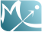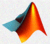# Simulation

## Simulation of a hierarchical model

In the What is a model? A joint probability distribution! section we proposed to consider a model as a joint probability distribution of random variables. Furthermore, the hierarchical structure of a model allows us to decompose this joint distribution into a product of conditional and marginal distributions.

Thus, if a model includes observations $\by$, individual parameters $\bpsi$, a design made up of observation times $\bt$ and source terms (e.g., doses) $\bu$, individual covariates $\bc$ and population parameters $\theta$, the joint distribution of these random variables can be written:

$$\pypsithcut(\by , \bpsi, \theta, \bu, \bc,\bt)=\pcypsiut(\by | \bpsi,\bu,\bt) \, \pcpsithc(\bpsi | \theta,\bc) \, \pth(\theta) \, \pc(\bc) \, \pu(\bu) \, \pt(\bt) .$$

Simulating this model means successively drawing the variables of the model using their associated submodels:

1. The population parameters $\theta$ from the distribution $\qth$.
2. The individual covariates $\bc$ from the distribution $\qc$.
3. The individual parameters $\bpsi$ from the distribution $\qcpsithc$ using the values of $\theta$ and $\bc$ obtained in steps 1 and 2.
4. The dose regimen $\bu$ from the distribution $\qu$.
5. The measurement times $\bt$ from the distribution $\qt$.
6. Lastly, observations $\by$ from the distribution $\qcypsiut$ using the values of $\bpsi$, $\bu$ and $\bt$ obtained at steps 3, 4 and 5.

Evidently, if certain variables are not considered random and are given, the associated simulation step is not performed.

We have already seen that for other tasks (model exploration, estimation) $\mlxtran$ is well-adapted to implementing this type of hierarchical model because it can deal with this structure by defining the various submodels in easy-to-read blocks. It is also possible to use $\mlxtran$ like a function from within R and Matlab. This capability means that we can write our own R or Matlab script in which the design is defined, the simulation function is called and the results are used for plotting figures, performing tests, etc. This solution combines the flexibility of the R and Matlab environments with the ability of $\mlxtran$ to easily encode complex models.

## Examples

The joint model ${\cal M}_2$ detailed in the Model selection section can be implemented with $\mlxtran$. We suppose that there can be either simple or multiple drug administrations either orally (adm=1) or by iv (adm=2). The model is "ready" to accept these different dose regimens, which are defined either within the data (for estimation) or the simulation script.MLXTran joint1_model.txt
[INDIVIDUAL]
input = {ka_pop,V_pop, Cl_pop,h0_pop,omega_ka, omega_V, omega_Cl,
omega_h0,beta_V,beta_Cl, weight}

EQUATION:
lw70=log(weight/70)

DEFINITION:
ka = {distribution=lognormal, reference=ka_pop, sd=omega_ka}
V  = {distribution=lognormal, reference=V_pop, sd=omega_V,
covariate=lw70, coefficient=beta_V}
Cl = {distribution=lognormal, reference=Cl_pop, sd=omega_Cl,
covariate=lw70, coefficient=beta_Cl}
h0 = {distribution=lognormal, reference=h0_pop, sd=omega_h0}

[OBSERVATION]
input = {ka, V, Cl, h0, gamma, a, b}

PK:

EQUATION:
ke=Cl/V
Cc  = Ac/V
h = h0*exp(gamma*Cc)

DEFINITION:
Concentration = {type=continuous, prediction=Cc, errorModel=combined1(a,b)}
Hemorrhaging  = {type=event, hazard=h}


Here is an example of a Matlab script that uses the joint1_model.txt model to simulate data for 10 patients. In this example, a dose of 50mg is administered every 24 hours over a 7 day period. The simulated data is stored in a cell array. We can then use exploredatapx for visualizing it.MATLAB

%% dose regimen

%% group
group1 = struct('size',10);

%% parameter
parameterName =  {'ka_pop','V_pop','Cl_pop','h0_pop','omega_ka','omega_V',...
'omega_Cl','omega_h0','beta_V','beta_Cl','gamma','a','b'};
parameterValue = [1, 8, 2, 0.01, 0.4, 0.2, 0.2, 0.1, 1, 0.75, 0.5, 0.1, 0.15];
param1 = struct('name',parameterName,'value',parameterValue);

%% output
outputName = {'Cc','h','Concentration','Hemorrhaging'};
outputTime = {0:1:200 , 0:1:200 , [1 2 4 6 12 18 28 52 76 100 124 148 172]};
output1 = struct('name',outputName,'time',outputTime);

'parameter',param1,'output',output1);

exploredatapx(data)


If we preferred, we could instead use R to run exactly the same simulations using exactly the same $\mlxtran$ model:

R

## dose regimen
admin1 <-list(adm = 1, amount = 50, time = seq(0, 144, by=24))

##group
group1 <- list(size=10)

## parameter
parameterName <-  c("ka_pop","V_pop","Cl_pop","h0_pop","omega_ka","omega_V",
"omega_Cl","omega_h0","beta_V","beta_Cl","gamma","a","b")
parameterValue <- c(1, 8, 2, 0.01, 0.4, 0.2, 0.2, 0.1, 1, 0.75, 0.5, 0.1, 0.15)
param1 <- list(name=parameterName, value=parameterValue)

## output
outputName <- c("Cc","h","Concentration","Hemorrhaging");
outputTime <- c(seq(0,200), seq(0,200), c(1 2 4 6 12 18 28 52 76 100 124 148 172))
output1 <- list(name=outputName, time=outputTime)

parameter=param1,output=output1);


It is also possible to include in the model a statistical model for the population parameters or the covariates (here, the weight) by adding sections [POPULATION] and/or [COVARIATE] to the $\mlxtran$ model. For example, we could add the following code to the previous $\mlxtran$ code:MLXTran joint2_model.txt
[POPULATION]
input = {V_0, Cl_0, gamma_V, gamma_Cl}

DEFINITION:
V_pop  = {distribution=lognormal,  reference=V_0, sd=gamma_V}
Cl_pop = {distribution=lognormal,  reference=Cl_0, sd=gamma_Cl}

[COVARIATE]
input = {omega_w}

DEFINITION:
weight  = {distribution=normal,  mean=70, sd=omega_w}


Remark

The simulix function and necessary R and Matlab connectors are still under development and will be available soon.